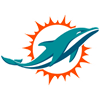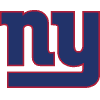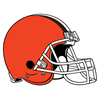DraftKings Miss Playoffs Odds
-10000
0.01 to 1Dolphins
50.0% implied probability

-10000
0.01 to 1Jaguars
50.0% implied probability

-5000
0.02 to 1Football Team
50.0% implied probability

-5000
0.02 to 1Giants
50.0% implied probability

-1000
0.1 to 1Panthers
50.0% implied probability

-1000
0.1 to 1Bears
50.0% implied probability

-600
0.17 to 1Eagles
50.0% implied probability

-500
0.2 to 1Patriots
50.0% implied probability

-500
0.2 to 1Broncos
50.0% implied probability

-400
0.25 to 1Steelers
50.0% implied probability

-400
0.25 to 1Falcons
50.0% implied probability

-400
0.25 to 1Seahawks
50.0% implied probability

-350
0.29 to 149ers
50.0% implied probability

-190
0.53 to 1Vikings
50.0% implied probability

-150
0.67 to 1Colts
50.0% implied probability

-110
0.91 to 1Raiders
50.0% implied probability

+120
1.2 to 1Saints
50.0% implied probability

+130
1.3 to 1Browns
50.0% implied probability

+155
1.55 to 1Chiefs
50.0% implied probability

+160
1.6 to 1Bengals
50.0% implied probability

+350
3.5 to 1Chargers
50.0% implied probability

+450
4.5 to 1Ravens
50.0% implied probability

+750
7.5 to 1Titans
50.0% implied probability

+800
8 to 1Cowboys
50.0% implied probability

+800
8 to 1Packers
50.0% implied probability

+1000
10 to 1Cardinals
50.0% implied probability

+1000
10 to 1Rams
50.0% implied probability

+1000
10 to 1Bills
50.0% implied probability

+1400
14 to 1Buccaneers
50.0% implied probability